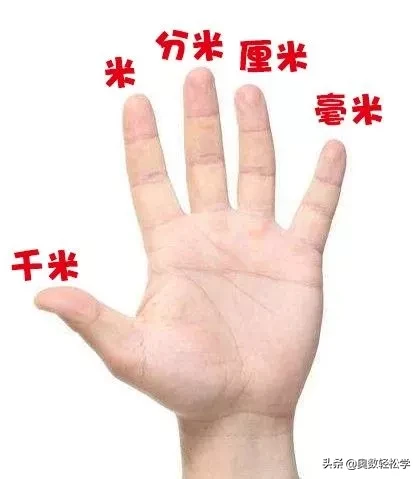# 一米等于多少平方（小学数学必考单位换算公式）## 长度单位

1千米（km）=1000米(m)，

1米(m)=10分米(dm)。

1米(m)=10分米(dm)=100厘米（cm）=1000毫米（mm）

1分米(dm)=10厘米(cm)=100毫米（mm）

1厘米(cm)=10毫米(mm）## 时间单位

1世纪=100年 1年=12个月

1日=24小时 1时=60分

：以地球自转周期为基准的时间单位，等于86400s。

：时间的辅助单位。

1分=60秒 1时=3600秒

：时间的基本单位。## 人民币单位

1元=10角

1角=10分

1元=100分## 重量单位

1吨=1000千克 1吨=1000 000克

：吨是重量单位，公制一吨等于1000公斤：计算船只容积的单位，一吨等于2.83立方米（合100立方英尺）。

1千克=1000克 500克=1斤

1千克=1公斤 1公斤=2斤

## 面积单位

1公顷=10000平方米（m²）

1平方米（m²）=100平方分米（dm²）

1平方分米（dm²）=100平方厘米（cm²）

1平方厘米（cm²）=100平方毫米（㎜²）## 体（容）积单位

1立方米=1000立方分米

1立方分米=1000立方厘米

1立方分米=1升

1立方厘米=1毫升

1立方米=1000升0.15千克=（ ）克

3.001吨=（ ）吨（ ）千克
3.7平方分米=（ ）平方毫米

5.80元=（ ）元（ ）角
( )分米=1.5米

( )吨( )千克=4.08吨
510米=( )千米

5米16厘米=( )米
5千克700克=( )千克

0.95米=( )厘米
4700米=( )千米

3650克=( )千克

40.06吨=( )千克

1.4平方米=( )分米

360平方米=( )公顷

7.05米＝（ ）米（ ）厘米

5.45千克＝（ ）千克（ ）克
3千米50米＝（ ）千米

（ ）时＝30分
3千克500克＝（ ）千克

（ ）时＝2时45分

2.78吨＝（ ）吨（ ）千克

0.25时＝（ ）分
504厘米＝（ ）米

4.2米＝（ ）米（ ）厘米
10米7分米＝（ ）米

0.06平方千米＝（ ）公顷
9千克750克＝（ ）千克

8.04吨＝（ ）吨（ ）千克

6.24平方米＝（ ）平方分米

2050m=（ ）km( )m

4.6吨=（ ）千克

（ ）㎡=750d㎡=( )c㎡

4650m=( )km

52公顷=（ ）平方米

3m³=( )dm³=( )cm³

6.3kg=( )g

2.4元=（ ）时（ ）分

6.05吨=（ ）千克

1050克=（ ）千克（ ）克

2.15小时=（ ）小时（ ）分

90秒=（ ）分

230平方分米=（ ）平方米

3.5平方千米=（ ）公顷

300立方分米=（ ）立方米

60毫米＝(　　　 )厘米

2吨＝(　　　 )千克

8米＝(　　　 )分米

5000克＝(　　　 )千克

3千克＝(　　　 )克

7千米＝(　　　 )米

400厘米＝(　　　 )米

6000千克＝(　　　 )吨

3吨500千克＝(　　　 )千克

3600千米＝(　　 )千米(　 )米

1吨－320千克＝(　　　 )千克

7008千克＝(　　 )吨(　　 )千克

4米7厘米＝(　　 )厘米

1米－54厘米＝(　　 )厘米

830克＋170克＝(　　)克＝( )千克

4.05立方分米=（ ）毫升

1.5时=（ ）分

1450毫升=（ ）升（ ）毫升

3.9升=（ ）毫升

1.25小时=（ ）小时（ ）分

8时40分=（ ）时

7040千克=（ ）吨（ ）千克

6km40m=( )km

3元5角=（ ）元

2.5公顷=（ ）平方米

8.05m³=( )dm³

3.06m³=( ) m³ ( ) dm³

3时15分=（ ）时

285秒=（ ）分

12吨60千克=（ ）吨

680mL=（ ）L

282 mL =（ ）cm³

0.04m=( )cm=( )mm

3250米=（ ）千米（ ）米

2 m³30 dm³ =（ ）m³

2.25时=（ ）时（ ）分

1．大树高17(　　　 )．

2．一只小猫重2(　　　 )．

3．火车每小时行78(　　　 )．

4．一辆坦克重6(　　　 )．

5．钥匙长60(　　　 )．

6．一个梨重320(　　　 )．

7．小华身高130(　　　 )．

1．1小袋水饺粉重5(　　　 )．

①克　 ②千克　 ③吨

2．数学课本的宽是145(　　　 )．

①毫米　 ②厘米　 ③分米

3．黄河牌汽车的载重量是7(　　　 )．

①克　 ②千克　 ③吨

4．武汉长江大桥比南京长江大桥短5102( 　 )．

①分米　 ②米　 ③千米

5．一个鸡蛋约重70(　　　 )．

①吨　 ②千克　 ③克

6．一支钢笔长14(　　　 )．

①分米　 ②厘米　 ③毫米

7．4千克○4千米．（ ）

①＝　 ②＜　 ③不能比较

8．两层楼之间有22个台阶，每个台阶的高度是15厘米，从一楼到四楼升高了(　　　 )．

①1320米　 ②990厘米　 ③1320厘米